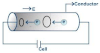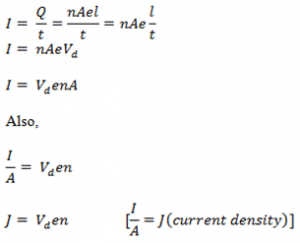## TyroCity

Physics XII Notes for Physics Notes

Posted on • Updated on

# Expression For Drift VelocityThe drift velocity is the average velocity that a particle, such as an electron, attains due to an electric field.
Consider a section of a metallic conductor of length l and cross-section area A. Let n be the no. of electrons per unit volume of the conductor. Then,
volume of the conductor = Al

no .of electrons in this volume = nAl

Total charge (Q) = nAel; where e is and electric charge.

On connecting a battery of potential V, Q charge flows through the conductor in time t, then current is: